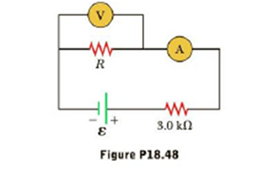Chapter 18, Problem 48AP

Chapter
Section
Textbook Problem

For the circuit shown in Figure P18.48, the voltmeter reads 6.0 V and the ammeter reads 3.0 m.A. Find (a) the value of R,(b) the emf of the battery and (c) the voltage across the 3.0-kΩ resistor.(d) What assumptions did you have to make to solve this problem?(a)

To determine
The ratio ΔVseriesΔVparallel .

Explanation

Formula to calculate the potential difference in series circuit is,

ΔVseries=IsR

• R is the resistance.
• Is is the current in series circuit.

Formula to calculate the potential difference in parallel circuit is,

ΔVparallel=IpR

• Ip is the current in series circuit

(b)

To determine
The ratio ΔPseriesΔPparallel .

Still sussing out bartleby?

Check out a sample textbook solution.

See a sample solution

The Solution to Your Study Problems

Bartleby provides explanations to thousands of textbook problems written by our experts, many with advanced degrees!

Get Started

Find more solutions based on key concepts